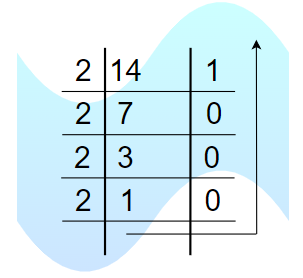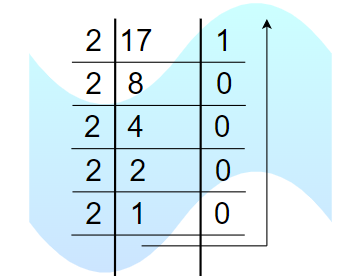Open In App

# How many types of number systems are there?

As Numbers are not only the mere symbols on a page but there is much more significance of numbers. In the study of mathematics, numerous number systems exist, each with its own significance and applications. From the familiar decimal system we use daily to the intriguing worlds of binary, octal, and hexadecimal, these systems offer unique perspectives on numerical representation. In this article, we explore the concepts of number systems and also learn about these different number system types.

The number system includes different types of numbers for example prime numbers, odd numbers, even numbers, rational numbers, whole numbers, etc. These numbers can be expressed in the form of figures as well as words accordingly. For example, the numbers like 40 and 65 expressed in the form of figures can also be written as forty and sixty-five.

## Numbers Definition

Numbers are used in various arithmetic values applicable to carry out various arithmetic operations like addition, subtraction, multiplication, etc which are applicable in daily lives for the purpose of calculation.

Numbers are the mathematical values or figures used for the purpose of measuring or calculating quantities. It is represented by numerals as 2, 4, 7, etc. Some examples of numbers are integers, whole numbers, natural numbers, rational and irrational numbers, etc.

The value of a number is determined by the digit, its place value in the number, and the base of the number system. Numbers generally also known as numerals are the mathematical values used for counting, measurements, labeling, and measuring fundamental quantities.

## What is a Number System?

A Number system or numeral system is defined as an elementary system to express numbers and figures. It is the unique way of representing of numbers in arithmetic and algebraic structure.

Thus, in simple words, the writing system for denoting numbers using digits or symbols in a logical manner is defined as a Number system. The numeral system Represents a useful set of numbers, reflects the arithmetic and algebraic structure of a number, and provides standard representation.

In the decimal number system, digits from 0 to 9 can be used to form all the numbers. With these digits, anyone can create infinite numbers. For example, 156,3907, 3456, 1298, 784859, etc. Other than digits, we can use alphabets such as A, B, C, D, E, and F (in Hexadecimal Number System) to represent different numbers.

## Types of Number Systems

Based on the base value and the number of allowed digits, number systems are of many types. The four common types of Number systems are:

• Decimal Number System
• Binary Number System
• Octal Number System

## Decimal Number System

A number system with a base value of 10 is termed a Decimal number system. It uses 10 digits i.e. 0-9 for the creation of numbers. Here, each digit in the number is at a specific place with a place value of a product of different powers of 10. Here, the place value is termed from right to left as the first place value called units, second to the left as Tens, so on Hundreds, Thousands, etc. Here, units have a place value of 100, tens have a place value of 101, hundreds as 102, thousands as 103, and so on.

For example, 12265 has place values as,

(1 × 104) + (2 × 103) + (2 × 102) + (6 × 101) + (5 × 100)

= (1 × 10000) + (2 × 1000) + (2 × 100) + (6 × 10) + (5 × 1)

= 10000 + 2000 + 200 + 60 + 5

= 12265

## Binary Number System

A number System with a base value of 2 is termed a Binary number system. It uses 2 digits i.e. 0 and 1 for the creation of numbers. The numbers formed using these two digits are termed Binary Numbers. The binary number system is very useful in electronic devices and computer systems because it can be easily performed using just two states ON and OFF i.e. 0 and 1.

Decimal Numbers 0-9 are represented in binary as 0, 1, 10, 11, 100, 101, 110, 111, 1000, and 1001

For example, 14 can be written as 1110, 19 can be written as 10011, and 50 can be written as 110010.

Example of 14 in the binary systemHere 14 can be written as 1110

## Octal Number System

Octal Number System is one in which the base value is 8. It uses 8 digits i.e. 0-7 for the creation of Octal Numbers. Octal Numbers can be converted to Decimal values by multiplying each digit with the place value and then adding the result. Here the place values are 80, 81, and 82. Octal Numbers are useful for the representation of UTF8 Numbers. Example,

(81)10 can be written as (121)8

(125)10 can be written as (175)8

A number System with a base value of 16 is known as Hexadecimal Number System. It uses 16 digits for the creation of its numbers. Digits from 0-9 are taken like the digits in the decimal number system but the digits from 10-15 are represented as A-F i.e. 10 is represented as A, 11 as B, 12 as C, 13 as D, 14 as E, and 15 as F. Hexadecimal Numbers are useful for handling memory address locations. Examples,

(185)10  can be written as (B9)16

(5440)10  can be written as (1540)16

(4265)10  can be written as (10A9)16

Other than these, one ancient number system which precedes the decimal number system is the Roman number system. Let’s learn about it in detail as follows:

## Roman Number System

The Roman numeral system is an ancient numerical system that was used in ancient Rome and throughout the Roman Empire. It is based on a combination of letters from the Latin alphabet to represent numbers. Here are the basic symbols used in the Roman numeral system along with their corresponding values:

### Rule of Roman Numeral

To write numbers in Roman numerals, we can use the following rules:

• The symbols I, X, C, and M can be repeated up to three times in a row.
• When a smaller value symbol appears before a larger value symbol, the smaller value is subtracted. For example, IV represents 4 (5 – 1) and IX represents 9 (10 – 1).
• When a smaller value symbol appears after a larger value symbol, the smaller value is added. For example, VI represents 6 (5 + 1) and XI represents 11 (10 + 1).

## Sample Problems on Types of Number System

Problem 1: Convert (4525)8 into a decimal.

Solution:

(4525)8 = 4 × 83 + 5 × 82 + 2 × 81 + 5 × 80

⇒ 45258 = 4 × 512 + 5 × 64 + 2 × 8 + 5 × 1

⇒ 45258 = 2048 + 320 + 16 + 5

⇒ 45258 = 238910

Problem 2: Convert (17)10 as a binary number.

Solution:Therefore (17)10 = (10001)2

Problem 3: Convert (1011110)2 into an octal number.

Solution:

Given (1011110)2 a binary number, to convert it into octal number

Using this table we can write give number as

001 011 110 i .e

001 = 1

011 = 3

110 = 6

So (1011110)2 in octal number is (136)8

Problem 4: Convert the Roman numeral XLVIII into its decimal equivalent.

Solution:

As we know, all numbers before the greatest symbol are subtracted from it and all the numbers after the greatest symbol are added,

XLVIII = 50 – 10 + 5 + 1 + 1 + 1 = 48

Thus, XLVIII is 48 in decimal representation.

Problem 5: Convert the Roman numeral MCCXLV into its decimal equivalent.

Solution:

As we know, all numbers before the greatest symbol are subtracted from it and all the numbers after the greatest symbol are added,

MCCXLV = 1000 + 100 + 100 – 10 + 50

= 1000 + 200 + 50 – 10

= 1240

So, the Roman numeral MCCXLV is equal to 1240 in the decimal number system.

## FAQs on Types of Number Systems

### Q1: Define Number System.

A number system or system of number is a way of representing and expressing numbers. It provides a set of symbols and rules for manipulating those symbols to perform arithmetic operations and solve mathematical problems.

### Q2: How many Types of Number Systems are there?

There are several types of number systems commonly used in mathematics and computer science. The most common ones are:

• Decimal Number System (base 10)
• Binary Number System (base 2)
• Octal Number System (base 8)

### Q3: How many Numerals are there in Binary Number System?

There are two numerals in binary number system i.e., 0 and 1.

### Q4: How many Numerals are there in Octal Number System?

There are ten numerals in decimal number system i.e., 0, 1, 2, 3, 4, 5, 6, and 7.

### Q5: How many numerals are there in Decimal Number System?

There are ten numerals in decimal number system i.e., 0, 1, 2, 3, 4, 5, 6, 7, 8, and 9.